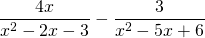Chapter 8: Rational Expressions

# 8.4 Addition and Subtraction of Rational Expressions

Adding and subtracting rational expressions is identical to adding and subtracting integers. Recall that, when adding fractions with a common denominator, you add the numerators and keep the denominator. This is the same process used with rational expressions. Remember to reduce the final answer if possible.

Example 8.4.1

Add the following rational expressions: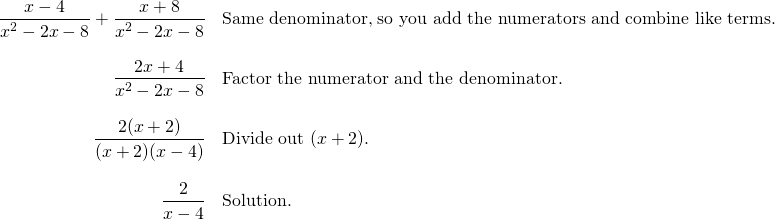Subtraction of rational expressions with a common denominator follows the same pattern, though the subtraction can cause problems if you are not careful with it. To avoid sign errors,  first distribute the subtraction throughout the numerator. Then treat it like an addition problem. This process is the same as “add the opposite,” which was seen when subtracting with negatives.

Example 8.4.2

Subtract the following rational expressions: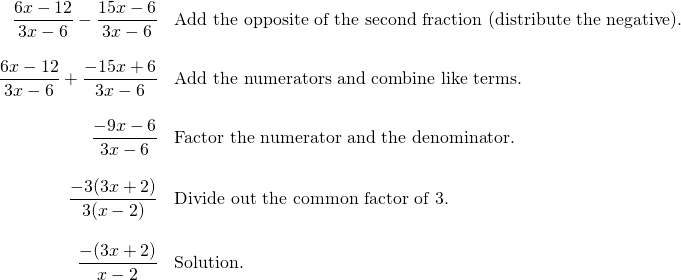When there is not a common denominator, first find the least common denominator (LCD) and alter each fraction so the denominators match.

Example 8.4.3

Add the following rational expressions: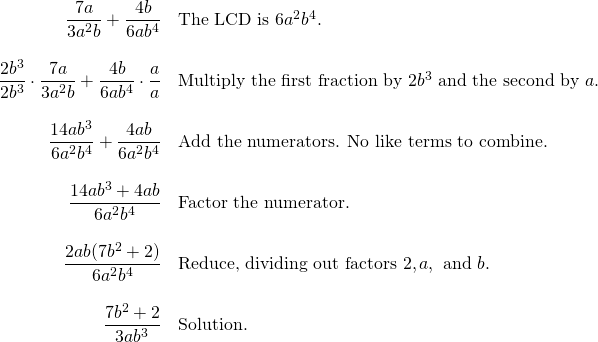Example 8.4.4

Subtract the following rational expressions: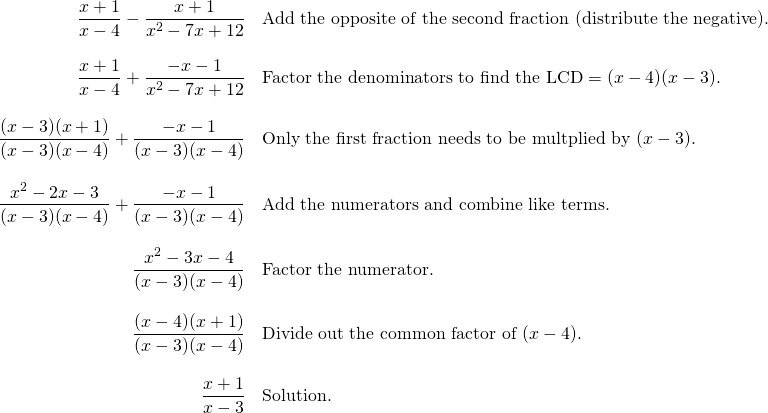# Questions

Add or subtract the rational expressions. Simplify your answers whenever possible.

1.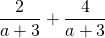2.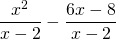3.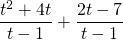4.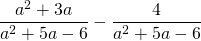5.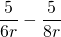6.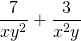7.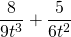8.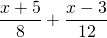9.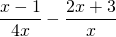10.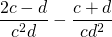11.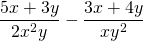12.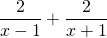13.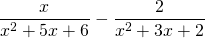14.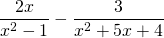15.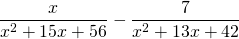16.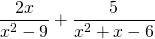17.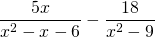18.